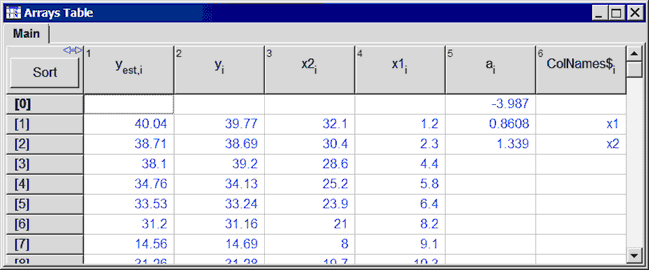Contents - Index

LinearRegression Procedure

The LinearRegression procedure (available in the Professional license) provides the parameters and statistics resulting from a linear regression curve fit to data contained in the Arrays or Lookup tables. The CURVEITFIT1D and CURVEFIT1DT procedures also provide this capability with one independent variable.  The LinearRegression procedure may have up to 100 independent variables.

The LinearRegression procedure provides capability similar to that provided with the LInear Regression menu command.  The differences are:

1.  The Linear Regression menu command is limited to 15 variables with a polynomial up to the 6th order for each variable.  The LinearRegression procedure allows up to 100 independent variables, but there is no polynomial expansion.  For example, if you need to fit Y as a function of X and X^2, the LinearRegression procedure will require separate columns of data for X and X^2.

2.  The LinearRegression procedure allows the parameters and statistics of the curve fitting process to be determined programmatically and thus they can be directly used in subsequent equations within the Equations window.

Format of the LinearRegression Procedure

Call LINEARREGRESSION(Table\$, Row1, RowN, DepVar\$, NInd, IndependVars\$[1..NInd],Exclude0, doUnits : a[0..NInd], rms, bias, R2, StdErr[0..NInd])

Inputs:

Table\$ is a string constant or string variable that contains the name of the table holding the data that are to be fit.  For data in the Lookup table, Table\$ is the name on the Lookup table tab.  For data in Arrays table, Table\$ must be the keyword 'ARRAYS' followed by a colon and the name of the tab of the Arrays table, e.g., 'ARRAYS:MAIN'.  Setting Table\$ to 'ARRAYS' is equivalent to setting it to 'ARRAYS:MAIN'.

Row1 is the first row in the table that is used in the curve fit.  It can be an integer or previously defined EES variable.

RowN is the last row in the table that is used in the curve fit.  It can be an integer or previously defined EES variable.

DepVar\$ is a string variable or string constant providing the name of column in the specified table for the dependent variable.  The column name is shown in the header cell of the column.  For variables in the Arrays table, is it not necessary to provide the [i] at the end of the array name.

Nind is the number of independent variables.  It can be an integer between 1 and 100 or a previously defined EES variable.

IndependentVars\$[1..NInd] is an array of NInd strings with each string being the name of a column in the specified table.

Exclude0 is either false# (0) or true# (1).  If this parameter is false, a constant, e.g., a will be determined in the curve fit process.  If this parameter is true, a will be set to zero.

DoUnits is optional.  If it is provided, it is either false# (0) or true# (1).  If the dependent and/or independent variables have units, the curve-fit parameters will also have associated units.  If DoUnits >0 then the units of each of the parameters will be determined.  If this input is not provided, the units will not be determined

Outputs:

a[0..NInd] is the array of curve fit parameters returned by the LINEARREGRESSION procedure.  Note that a is returned as 0 is Exclude0 is true# and must be included in the array.

All following outputs are optional.

RMS is root mean square error defined as: sqrt((1/N)*sum[(Y-Y`)^2] where N is the number of data points, and Y` is the estimated value of Y, where Y is the dependent variable.

BIAS is the bias error defined as: (1/N)*sum[(Y-Y`)].

R|2 is the correlation coefficient between dependent and independent variables.

StdErr[0..NInd] is the standard error of the curve fit parameters defined as the square root of the estimated variance of the parameter

Example:

x1[1..8]=[1.2,2.3,4.4,5.8,6.4,8.2,9.1,10.3]

x2[1..8]=[32.1,30.4,28.6,25.2,23.9,21,8,19.7]

y[1..8]=[39.77,38.69,39.2,34.13,33.24,31.16,14.69,31.28]

NInd=2

ColNames\$[1..NInd]=['x1','x2']

Call linearregression('Arrays',1,8,'y',NInd,ColNames\$[1..NInd],false#,false#: a[0..NInd],rms,bias,R2)

Duplicate i=1,8

y_est[i]=a+a*x1[i]+a*x2[i]

End

After solving, the Arrays table will show the following values for the a[ ] array.